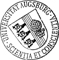## Asymptotic Goodness-of-fit Tests for the Palm Mark Distribution of Stationary Point Processes with Correlated Marks

• We consider spatially homogeneous marked point patterns in an unboundedly expanding convex sampling window. Our main objective is to identify the distribution of the typical mark by constructing an asymptotic chi-square-goodness-of-fit test. The corresponding test statistic is based on a natural empirical version of the Palm mark distribution and a smoothed covariance estimator which turns out to be mean-square consistent. Our approach does not require independent marks and allows dependences between the mark field and the point pattern. Instead we impose a suitable beta-mixing condition on the underlying stationary marked point process which can be checked for a number of Poisson-based models and, in particular, in the case of geostatistical marking. Our method needs a central limit theorem for beta-mixing random fields which is proved by extending Bernstein's blocking technique to non-cubic index sets and seems to be of interest in its own right. By large-scale model-basedWe consider spatially homogeneous marked point patterns in an unboundedly expanding convex sampling window. Our main objective is to identify the distribution of the typical mark by constructing an asymptotic chi-square-goodness-of-fit test. The corresponding test statistic is based on a natural empirical version of the Palm mark distribution and a smoothed covariance estimator which turns out to be mean-square consistent. Our approach does not require independent marks and allows dependences between the mark field and the point pattern. Instead we impose a suitable beta-mixing condition on the underlying stationary marked point process which can be checked for a number of Poisson-based models and, in particular, in the case of geostatistical marking. Our method needs a central limit theorem for beta-mixing random fields which is proved by extending Bernstein's blocking technique to non-cubic index sets and seems to be of interest in its own right. By large-scale model-based simulations the performance of our test is studied in dependence of the model parameters which determine the range of spatial correlations.• Dokument_1.pdfAuthor: Lothar HeinrichGND, Sebastian Lück, Volker Schmidt urn:nbn:de:bvb:384-opus4-12082 https://opus.bibliothek.uni-augsburg.de/opus4/1499 Preprints des Instituts für Mathematik der Universität Augsburg (2011-06) Preprint English Universität Augsburg Institute of Stochastics, Ulm University 2011/03/21 geglaettete Kovarianzschätzung; absolute Regularität; faktorielle Momentenmasseempirical Palm mark distribution; reduced factorial moment measures; central limit theorem; beta-mixing marked point process Markierter Punktprozess; Anpassungstest; Zentraler Grenzwertsatz Mathematisch-Naturwissenschaftlich-Technische Fakultät Mathematisch-Naturwissenschaftlich-Technische Fakultät / Institut für Mathematik Mathematisch-Naturwissenschaftlich-Technische Fakultät / Institut für Mathematik / Lehrstuhl für Stochastik und ihre Anwendungen 5 Naturwissenschaften und Mathematik / 51 Mathematik / 510 MathematikDeutsches Urheberrecht mit Print on Demand